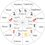# Estimate of expected loss on the portfolio

A portfolio consists of two (long) assets £100 million each. The probability of default over the next year is 10% for the first asset, 20% for the second asset, and the joint probability of default is 3%. Estimate the expected loss on this portfolio due to credit defaults over the next year assuming zero recovery rate.

Answer to this question is £32million. I don't understand how it is computed? What is the logic behind this answer?

Would any quant finance analyst of Brilliant.org explain it?

Answer:- There are two events. Event 1) First asset defaults over the next year. Event 2) Second asset defaults over the next year. Total worth of portfolio is £200 million.

The probability that the first asset defaults over the next year is 10% of £100 million= £10million. ...(1)

The probability that the second asset defaults over the next year is 20% of £100 million= £20 million....(2)

The joint probability of both these events occurring simultaneously over the next year is 3% of £200 million= £6million....(3).

But it include the probability of both the events occuring jointly and independently =2%of £200 million= £4 million....(4). which should be deducted from (1)+(2)+(3)= £36million. So (1)+(2)+(3)-(4)= £32 millionNote by Winod Dhamnekar
1 year, 9 months ago

This discussion board is a place to discuss our Daily Challenges and the math and science related to those challenges. Explanations are more than just a solution — they should explain the steps and thinking strategies that you used to obtain the solution. Comments should further the discussion of math and science.

When posting on Brilliant:

• Use the emojis to react to an explanation, whether you're congratulating a job well done , or just really confused .
• Ask specific questions about the challenge or the steps in somebody's explanation. Well-posed questions can add a lot to the discussion, but posting "I don't understand!" doesn't help anyone.
• Try to contribute something new to the discussion, whether it is an extension, generalization or other idea related to the challenge.

MarkdownAppears as
*italics* or _italics_ italics
**bold** or __bold__ bold
- bulleted- list
• bulleted
• list
1. numbered2. list
1. numbered
2. list
Note: you must add a full line of space before and after lists for them to show up correctly
paragraph 1paragraph 2

paragraph 1

paragraph 2

[example link](https://brilliant.org)example link
> This is a quote
This is a quote
    # I indented these lines
# 4 spaces, and now they show
# up as a code block.

print "hello world"
# I indented these lines
# 4 spaces, and now they show
# up as a code block.

print "hello world"
MathAppears as
Remember to wrap math in $$ ... $$ or $ ... $ to ensure proper formatting.
2 \times 3 $2 \times 3$
2^{34} $2^{34}$
a_{i-1} $a_{i-1}$
\frac{2}{3} $\frac{2}{3}$
\sqrt{2} $\sqrt{2}$
\sum_{i=1}^3 $\sum_{i=1}^3$
\sin \theta $\sin \theta$
\boxed{123} $\boxed{123}$